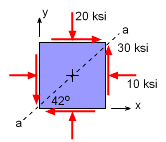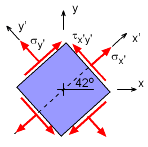Ch 7. Stress Analysis Multimedia Engineering Mechanics PlaneStress PrincipalStresses Mohr's Circlefor Stress Failure PressureVessels
 Chapter 1. Stress/Strain 2. Torsion 3. Beam Shr/Moment 4. Beam Stresses 5. Beam Deflections 6. Beam-Advanced 7. Stress Analysis 8. Strain Analysis 9. Columns Appendix Basic Math Units Basic Equations Sections Material Properties Structural Shapes Beam Equations Search eBooks Dynamics Fluids Math Mechanics Statics Thermodynamics Author(s): Kurt Gramoll ©Kurt GramollMECHANICS - EXAMPLEStress Element Example What is the shear stress on a plane 42o from the horizontal (plane a-a)? SolutionStress Element Rotated 42o The stress state at other orientations can be determined using the stress rotation equations. This problems asks for the shear stress on a plane 42o from the horizontal. The basic parameters are      σx = -10 ksi      σy = -20 ksi      τxy = 30 ksi      θ = 42o Notice, the two normal stress are negative since the arrows in the original problem diagram are pointing in the negative direction. Using the shear stress rotation equation gives,      τx´y´ = - [(σx -σy) sin 2θ] / 2 + τxy cos 2θ            = -[(-10 -(-20)) sin84] / 2 + 30 cos84            = -4.973 + 3.136 ksi      τx'y' = -1.837 ksi

Practice Homework and Test problems now available in the 'Eng Mechanics' mobile app
Includes over 400 problems with complete detailed solutions.
Available now at the Google Play Store and Apple App Store.